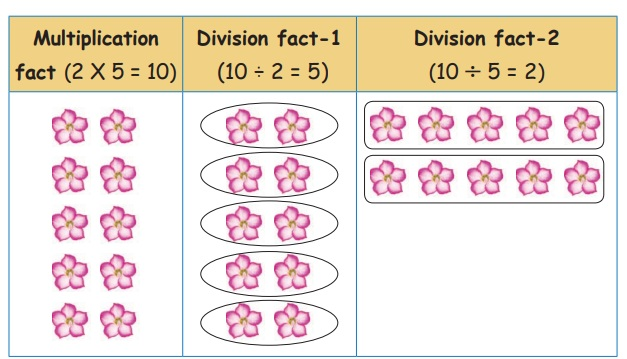Home | | Maths 3rd Std | Multiplication and Division

# Multiplication and Division

Construct the division fact for the multiplication tables

Multiplication and Division

Consider the division fact

8 ÷ 2 = 4

Roja has 8 chocolates. She divides them among 2 of her friends. How many chocolates would each friend get?

This means 2 groups of 4 make 8 which can be written as 2 × 4 = 8It means multiplication and division are reverse operations.

2 × 4 = 8

8 ÷ 2 = 4

Consider the arrangement of these 10 flowers.5 groups of 2 flowers make 10 flowers 5 × 2 = 10

2 groups of 5 flowers make 10 flowers 2 × 5 = 10

10 flowers can be put into 5 groups of 2 flowers each 10 ÷ 5 = 2

10 flowers can be put into 2 groups of 5 flowers each 10 ÷ 2 = 5

Finding the division fact for the given multiplication fact.Construct the division fact for the multiplication tables 3.Construct the division fact for the multiplication tables 4.Construct the division fact for the multiplication tables 5.Construct the division fact for the multiplication tables 10.Find the quotient of the following.

1. 12 ÷ 4 = 3

4 × 1= 4

4 × 2 = 8

4 ×3 =12

Say multiplication table 4 till you get product 12.

3 times 4 is 12 i.e., 3 × 4 = 12

Hence 12 ÷ 4 = 3

2. 25 ÷ 5 = 5

5 × 1 = 5

5 × 2 = 10

5 × 3 = 15

5 × 4 = 20

5 × 5 = 25

Say multiplication table 5 till you get product 25

5 times 5 is 25 i.e.,5 × 5 = 25

Hence 25 ÷ 5 = 5

Tags : Numbers | Term 3 Chapter 2 | 3rd Maths , 3rd Maths : Term 3 Unit 2 : Numbers
Study Material, Lecturing Notes, Assignment, Reference, Wiki description explanation, brief detail
3rd Maths : Term 3 Unit 2 : Numbers : Multiplication and Division | Numbers | Term 3 Chapter 2 | 3rd Maths